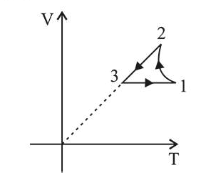# Which of the following is an equivalent cyclic process corresponding to the thermodynamic cyclic given in the figure?

Question:

Which of the following is an equivalent cyclic process corresponding to the thermodynamic cyclic given in the figure?

where, $1 \rightarrow 2$ is adiabatic.

(Graphs are schematic and are not to scale)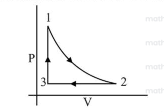1.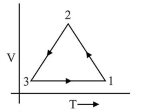2.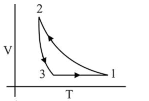3.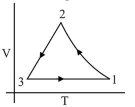4.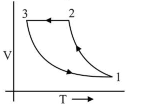Correct Option: , 3

Solution:

(3) For process $3 \rightarrow 1$ volume is constant

$\therefore \quad$ Graph given in option (4) is wrong.

And process $1 \rightarrow 2$ is adiabatic $\therefore$ graph in option (1) is wrong

$\because \quad v=$ constant

$P \uparrow, T \uparrow$

For Process $2 \rightarrow 3$ Pressure constant i.e., $P=$ constant

$\therefore \quad V \downarrow T \downarrow$

Hence graph (3) is the correct $V-T$ graph of given $P-V$ graph